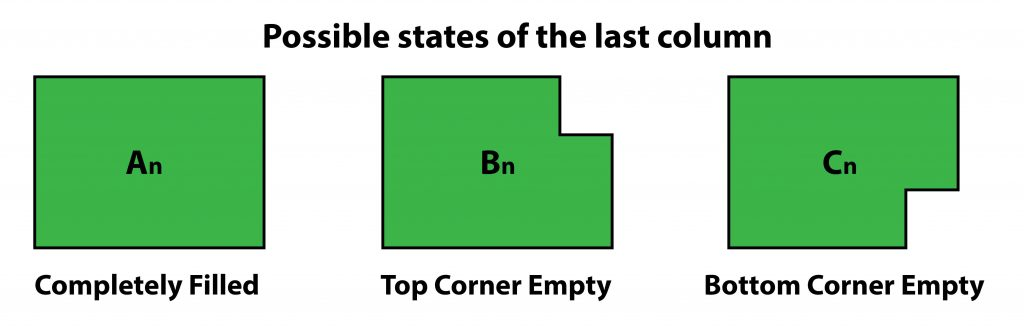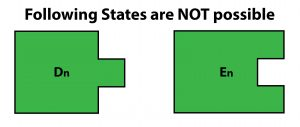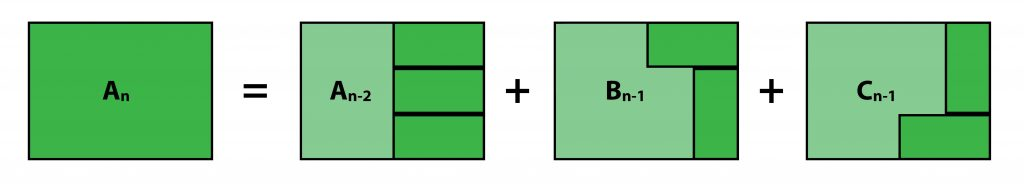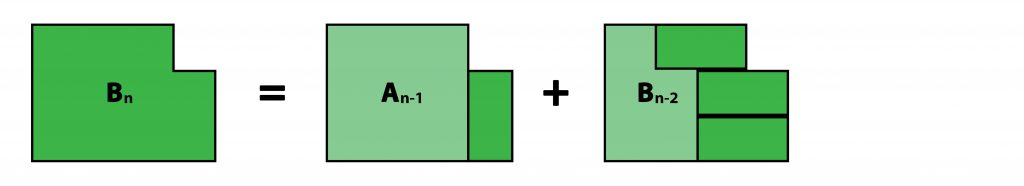Related Articles

# Tiling with Dominoes

• Difficulty Level : Hard
• Last Updated : 05 Jun, 2018

Given a 3 x n board, find the number of ways to fill it with 2 x 1 dominoes.

Example 1
Following are all the 3 possible ways to fill up a 3 x 2 board.Example 2
Here is one possible way of filling a 3 x 8 board. You have to find all the possible ways to do so.Examples :

Input : 2
Output : 3

Input : 8
Output : 153

Input : 12
Output : 2131


## Recommended: Please try your approach on {IDE} first, before moving on to the solution.

Defining Subproblems:
At any point while filling the board, there are three possible states that the last column can be in:An =  No. of ways to completely fill a 3 x n board. (We need to find this)
Bn =  No. of ways to fill a 3 x n board with top corner in last column not filled.
Cn =  No. of ways to fill a 3 x n board with bottom corner in last column not filled.


Note: The following states are impossible to reach:Finding Reccurences
Note: Even though Bn and Cn are different states, they will be equal for same ‘n’. i.e Bn = Cn
Hence, we only need to calculate one of them.

Calculating An:Calculating Bn:Final Recursive Relations are:Base Cases:## C++

 // C++ program to find no. of ways// to fill a 3xn board with 2x1 dominoes.#include using namespace std;  int countWays(int n){    int A[n + 1], B[n + 1];    A = 1, A = 0, B = 0, B = 1;    for (int i = 2; i <= n; i++) {        A[i] = A[i - 2] + 2 * B[i - 1];        B[i] = A[i - 1] + B[i - 2];    }      return A[n];}  int main(){    int n = 8;    cout << countWays(n);    return 0;}

## Java

 // Java program to find no. of ways// to fill a 3xn board with 2x1 dominoes.import java.io.*;  class GFG {      static int countWays(int n)    {        int []A = new int[n+1];        int []B = new int[n+1];        A = 1; A = 0;        B = 0; B = 1;        for (int i = 2; i <= n; i++)         {            A[i] = A[i - 2] + 2 * B[i - 1];            B[i] = A[i - 1] + B[i - 2];        }              return A[n];    }      // Driver code    public static void main (String[] args)     {        int n = 8;        System.out.println(countWays(n));    }}  // This code is contributed by anuj_67.

## Python 3

 # Python 3 program to find no. of ways# to fill a 3xn board with 2x1 dominoes.  def countWays(n):      A =  * (n + 1)    B =  * (n + 1)    A = 1    A = 0    B = 0    B = 1    for i in range(2, n+1):        A[i] = A[i - 2] + 2 * B[i - 1]        B[i] = A[i - 1] + B[i - 2]          return A[n]  n = 8print(countWays(n))  # This code is contributed by Smitha

## C#

 // C# program to find no. of ways// to fill a 3xn board with 2x1 dominoes.using System;  class GFG {      static int countWays(int n)    {        int []A = new int[n+1];        int []B = new int[n+1];        A = 1; A = 0;        B = 0; B = 1;        for (int i = 2; i <= n; i++)         {            A[i] = A[i - 2] + 2 * B[i - 1];            B[i] = A[i - 1] + B[i - 2];        }              return A[n];    }      // Driver code    public static void Main ()     {        int n = 8;        Console.WriteLine(countWays(n));    }}  // This code is contributed by anuj_67.

## PHP

 
Output :
153My Personal Notes arrow_drop_up